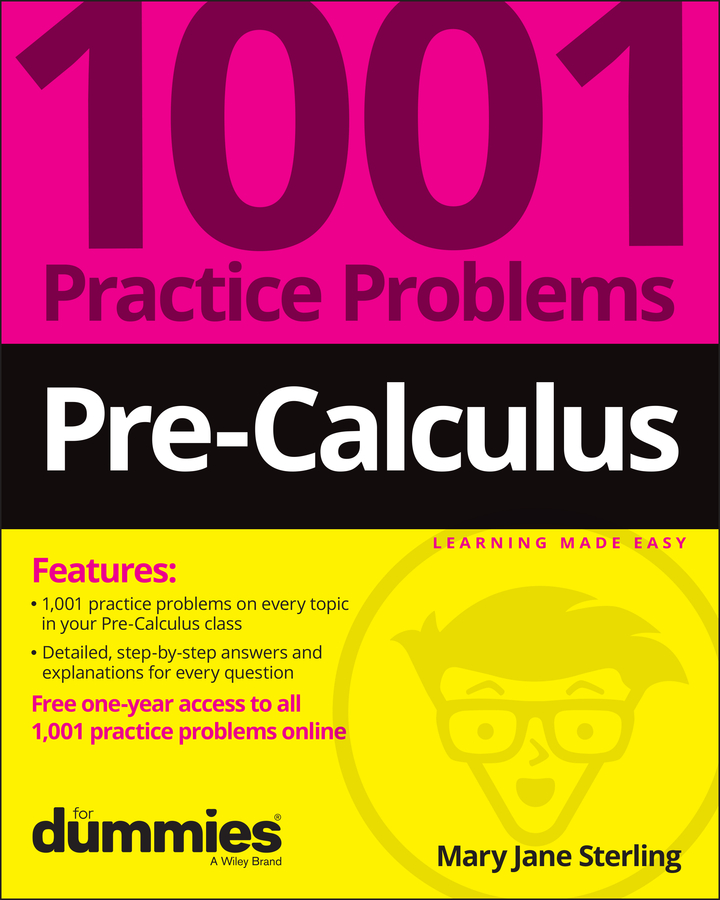##### Pre-Calculus: 1001 Practice Problems For Dummies (+ Free Online Practice)You can use the rational root theorem to narrow down the search for roots of polynomials. While Descartes’s rule of signs only narrows down the real roots into positive and negative, the rational root theorem says that some real roots are rational (they can be expressed as a fraction). It also helps you create a list of the possible rational roots of any polynomial.

The problem? Not every root is rational, because some may be irrational. A polynomial may even have only irrational roots. But this theorem is always the next place to start in your search for roots; it will at least give you a diving-off point. Besides, the problems you’re presented with in pre-calculus more than likely have at least one rational root!

Follow these general steps to ensure that you find every root:

1. Use the rational root theorem to list all possible rational roots.

2. Pick one root from the list in Step 1 and use long division or synthetic division to find out if it is, in fact, a root.

1. If the root doesn’t work, try another guess.

2. If the root works, proceed to Step 3. (Otherwise, keep trying more possible rational roots, until you either find one — or you run out of possibilities!)

3. Using the depressed polynomial (the one you get after doing the synthetic division in Step 2), test the root that worked to see if it works again.

1. If it works, repeat Step 3 again.

2. If it doesn’t work, return to Step 2 and try a different root from the list in Step 1.

4. List all the roots you find that work. You have now found all rational roots.

Note: If your remaining depressed polynomial is quadratic, you can use the quadratic formula to find the two remaining roots. These roots will either be irrational or have an imaginary component.